# SSAT Upper Level Math : How to find out if a point is on a line with an equation

## Example Questions

← Previous 1

### Example Question #1 : How To Find Out If A Point Is On A Line With An Equation

Which of the following points is on both the line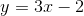and the line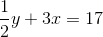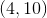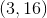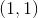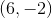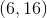Explanation:

In the multiple choice format, you can just plug in these points to see which satisfies both equations.andwork for the first but not the second, andandwork for the second but not the first. Onlyworks for both.

Alternatively (or if you were in a non-multiple choice scenario), you could set the equations equal to each other and solve for one of the variables. So, for instance,and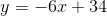so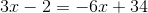Now you can solve and get. Plug this back into either of the original equations and get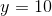.

### Example Question #2 : How To Find Out If A Point Is On A Line With An Equation

A line has the equation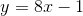. Which of the following points lies on the line?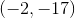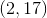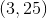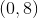Explanation:

Plug the x-coordinate of an answer choice into the equation to see if the y-coordinate matches with what comes out of the equation.

For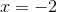,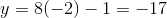### Example Question #3 : How To Find Out If A Point Is On A Line With An Equation

Which of the following points lies on the line with equation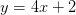?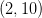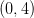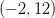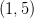Explanation:

To find which point lies on the line, plug in the x-coordinate value of an answer choice into the equation. If the y-coordinate value that comes out of the equation matches that of the answer choice, then the point is on the line.

For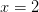,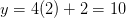So then,lies on the line.

### Example Question #4 : How To Find Out If A Point Is On A Line With An Equation

Which of the following points lies on the line with the equation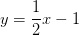?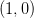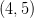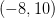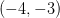Explanation:

To find if a point is on the line, plug in the x-coordinate of the answer choice into the given equation. If the resulting value for the y-coordinate matches that of the answer choice, then that point is on the line.

For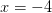,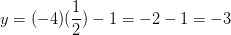### Example Question #5 : How To Find Out If A Point Is On A Line With An Equation

Which of the following points lies on the line with the equation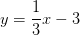?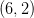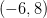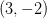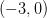Explanation:

To find if a point is on the line, plug in the x-coordinate of the answer choice into the given equation. If the resulting value for the y-coordinate matches that of the answer choice, then that point is on the line.

For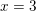,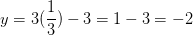### Example Question #6 : How To Find Out If A Point Is On A Line With An Equation

Which of the following points lies on the line with the equation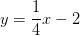?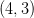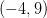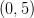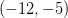Explanation:

To find if a point is on the line, plug in the x-coordinate of the answer choice into the given equation. If the resulting value for the y-coordinate matches that of the answer choice, then that point is on the line.

For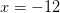,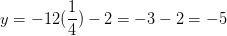### Example Question #7 : How To Find Out If A Point Is On A Line With An Equation

Which of the following points lies on the line with the equation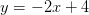?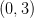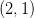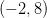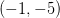Explanation:

To find if a point is on the line, plug in the x-coordinate of the answer choice into the given equation. If the resulting value for the y-coordinate matches that of the answer choice, then that point is on the line.

For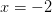,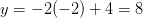### Example Question #8 : How To Find Out If A Point Is On A Line With An Equation

Which of the following points is on the line with the equation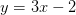?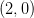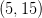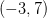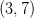Explanation:

To find if a point is on the line, plug in the x-coordinate of the answer choice into the given equation. If the resulting value for the y-coordinate matches that of the answer choice, then that point is on the line.

For,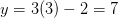### Example Question #51 : Coordinate Geometry

Consider the lines described by the following two equations:

4y = 3x2

3y = 4x2

Find the vertical distance between the two lines at the points where x = 6.

21

48

36

44

12

21

Explanation:

Since the vertical coordinates of each point are given by y, solve each equation for y and plug in 6 for x, as follows:Taking the difference of the resulting -values give the vertical distance between the points (6,27) and (6,48), which is 21.

### Example Question #52 : Coordinate Geometry

For the line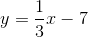Which one of these coordinates can be found on the line?

(3, 7)

(3, 6)

(6, 12)

(6, 5)

(9, 5)

(3, 6)

Explanation:

To test the coordinates, plug the x-coordinate into the line equation and solve for y.

y = 1/3x -7

Test (3,-6)

y = 1/3(3) – 7 = 1 – 7 = -6   YES!

Test (3,7)

y = 1/3(3) – 7 = 1 – 7 = -6  NO

Test (6,-12)

y = 1/3(6) – 7 = 2 – 7 = -5  NO

Test (6,5)

y = 1/3(6) – 7 = 2 – 7 = -5  NO

Test (9,5)

y = 1/3(9) – 7 = 3 – 7 = -4  NO

← Previous 1